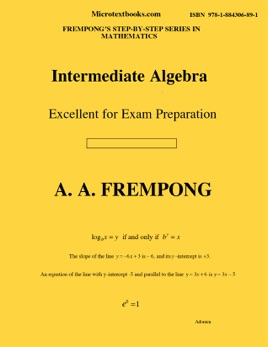• \$11.99

## Publisher Description

Intermediate Algebra is a very user-friendly mathematics book, and covers the following topics: real number operations; exponents ; radicals; fractional exponents; factoring polynomials; solving quadratic equations and applications; graphs, slopes, intercepts, and equations of straight lines; graphs of parabolas; linear inequalities; compound inequalities; inequality word problems; reduction, multiplication, division, and addition of algebraic fractions; solving fractional or rational equations; solving radical equations; variation and variation problems. complex numbers; square roots of negative numbers; addition, multiplication and division of complex numbers; absolute value equations; absolute value inequalities; logarithms; logarithmic equations and exponential equations; graphs of exponential and logarithmic functions; applications of exponential and logarithmic functions; one-to-one functions; composite functions, inverse functions and inverse relations.